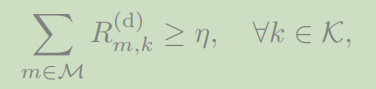# Convex programming error

i am new at CVX. this problem is convex and i stimulate it ，the result shows convex programing error. plz somebody help me that where is my problem(or give me some advice). thanks sincerely！

``````clc
clear all

H = 120;Ws = [0,0];Wn = [300,300];Wf = [500,500];Wr = [400,400];
Pt = 4; B0 = 10^(-3);a = 2;N = 10^(-10);
ksr = 0.01;krn = 0.01;krf = 0.01;

dsr = sqrt(H^2+norm(Wr-Ws)^2);
drn = sqrt(H^2+norm(Wr-Wn)^2);
drf = sqrt(H^2+norm(Wr-Wf)^2);

hsr = B0/dsr^(-a);
hrn = B0/drn^(-a);
hrf = B0/drf^(-a);

b1 = 1+ksr^2; b2 = 1+krf^2; b3 = 1+krn^2;
d1 = (ksr^2+krf^2+ksr^2*krf^2);
d2 = (ksr^2+krn^2+ksr^2*krn^2);

cvx_begin
variable Ps
variable Pr
variable an
variable af
variable haha
maximize haha;
subject  to
0.5*log2(1+an*Ps/N*hsr^2*Pr/N*hrn^2/(d2*Ps/N*hsr^2 ...
*Pr/N*hrn^2+b1*Ps/N*hsr^2+b3*Pr/N*hrn^2+1))  + ...
0.5*log2(1+af*Ps/N*hsr^2*Pr/N*hrf^2/((an+d1)*Ps/N*hsr^2 ...%R1 = log2(1+SINR)
*Pr/N*hrf^2+b1*Ps/N*hsr^2+b2*Pr/N*hrf^2+1)) >= haha;%sum(R1 + R2) >= haha
0 < an;
an < 1;
0< af;
af < 1;
an + af == 1;
Ps + Pr == Pt;
Ps >= 0;
Pr >= 0;
Rn >= 1;
Rf >= 1;%objective function and constraints are convex
``````

cvx_end

The first constraint has many CVX rule violations. Have you proven that this constraint is convex, i.e, that the left-hand side is concave?

1 Like

thanks very much. the paper already proven that the left constraints is joint concaveShow us the proof. Is it constructive, in a manner consistent with CVX’s rules?